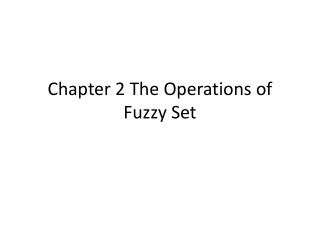DownloadDownload PresentationChapter 2 The Operations of Fuzzy Set

# Chapter 2 The Operations of Fuzzy Set

Download Presentation## Chapter 2 The Operations of Fuzzy Set

- - - - - - - - - - - - - - - - - - - - - - - - - - - E N D - - - - - - - - - - - - - - - - - - - - - - - - - - -
##### Presentation Transcript

1. Chapter 2 The Operations of Fuzzy Set

2. Outline • Standard operations of fuzzy set • Fuzzy complement • Fuzzy union • Fuzzy intersection • Other operations in fuzzy set • Disjunctive sum • Difference • Distance • Cartesian product • T-norms and t-conorms

3. Standard operation of fuzzy set • Complement

4. Standard operation of fuzzy set • Intersection

5. Fuzzy complement • C:[0,1][0,1]

6. Fuzzy complement

7. Fuzzy complement • Axioms C1 and C2 called “axiomatic skeleton ” are fundamental requisites to be a complement function, i.e., for any function C:[0,1][0,1] that satisfies axioms C1 and C2 is called a fuzzy complement. • Additional requirements

8. Fuzzy complement • Example 1: Standard function • Axiom C1 • Axiom C2 • Axiom C3 • Axiom C4

9. Fuzzy complement • Example 2: • Axiom C1 • Axiom C2 • Axiom C3 • Axiom C4

10. Fuzzy complement • Example 3: • Axiom C1 • Axiom C2 • Axiom C3 • Axiom C4

11. Fuzzy complement • Example 4: Yager’s function • Axiom C1 • Axiom C2 • Axiom C3 • Axiom C4

12. Fuzzy complement • Fuzzy partition If m subsets are defined in X, m-tuple (A1, A2,…,Am) holding the following conditions is called a fuzzy partition.

13. Fuzzy union

14. Fuzzy union • Axioms U1 ,U2,U3 and U4 called “axiomatic skeleton ” are fundamental requisites to be a union function, i.e., for any function U:[0,1]X[0,1][0,1] that satisfies axioms U1,U2,U3 and U4 is called a fuzzy union. • Additional requirements

15. Fuzzy union • Example 1: Standard function • Axiom U1 • Axiom U2 • Axiom U3 • Axiom U4 • Axiom U5 • Axiom U6

16. Fuzzy union • Example 2: Yager’s function • Axiom U1 • Axiom U2 • Axiom U3 • Axiom U4 • Axiom U5 • Axiom U6

17. Fuzzy union

18. Fuzzy union • Some frequently used fuzzy unions • Probabilistic sum (Algebraic Sum): • Bounded Sum (Bold union): • Drastic Sum: • Hamacher’s Sum

19. Fuzzy union

20. Fuzzy intersection

21. Fuzzy intersection • Axioms I1 ,I2,I3 and I4 called “axiomatic skeleton ” are fundamental requisites to be a intersection function, i.e., for any function I:[0,1]X[0,1][0,1] that satisfies axioms I1,I2,I3 and I4 is called a fuzzy intersection. • Additional requirements

22. Fuzzy intersection • Example 1: Standard function • Axiom I1 • Axiom I2 • Axiom I3 • Axiom I4 • Axiom I5 • Axiom I6

23. Fuzzy intersection • Example 2: Yager’s function • Axiom I1 • Axiom I2 • Axiom I3 • Axiom I4 • Axiom I5 • Axiom I6

24. Fuzzy intersection

25. Fuzzy intersection • Some frequently used fuzzy intersections • Probabilistic product (Algebraic product): • Bounded product (Bold intersection): • Drastic product : • Hamacher’s product

26. Fuzzy intersection

27. Other operations • Disjunctive sum (exclusive OR)

28. Other operations

29. Other operations

30. Other operations • Disjoint sum (elimination of common area)

31. Other operations • Difference • Crisp set • Fuzzy set : Simple difference By using standard complement and intersection operations. • Fuzzy set : Bounded difference

32. Other operations • Example • Simple difference

33. Other operations • Example • Bounded difference

34. Other operations • Distance and difference

35. Other operations • Distance • Hamming distance • Relative Hamming distance

36. Other operations • Euclidean distance • Relative Euclidean distance • Minkowski distance (w=1-> Hamming and w=2-> Euclidean)

37. Other operations • Cartesian product • Power • Cartesian product

38. Other operations • Example: • A = { (x1, 0.2), (x2, 0.5), (x3, 1) } • B = { (y1, 0.3), (y2, 0.9) }

39. t-norms and t-conorms (s-norms)

40. t-norms and t-conorms (s-norms)

41. t-norms and t-conorms (s-norms) • Duality of t-norms and t-conorms • Applying complements • DeMorgan’s law